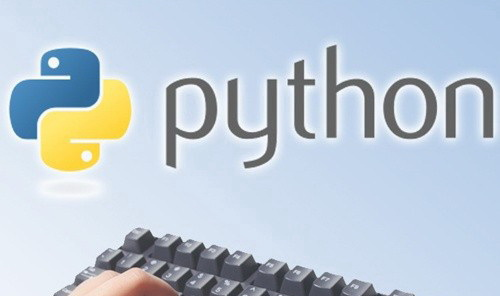2016
09-06

# Python中多线程及程序锁浅析

```# coding : uft-8
__author__ = 'Phtih0n'

def __init__(self):

def run(self):
global n
print n
n += 1

if "__main__" == __name__:
n = 0
for i in range(0, 10):
t.start()
t.join```

```# coding : uft-8
__author__ = 'Phtih0n'

def __init__(self):

def run(self):
global n, lock
time.sleep(1)
if lock.acquire():
print n , self.name
n += 1
lock.release()

if "__main__" == __name__:
n = 1
for i in range(1, 200):
t.start()
t.join()```